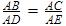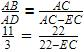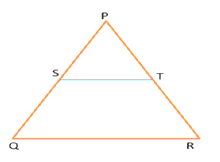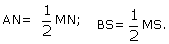Courses

# Test: Converse Of BPT

## 10 Questions MCQ Test Mathematics (Maths) Class 10 | Test: Converse Of BPT

Description
This mock test of Test: Converse Of BPT for Class 10 helps you for every Class 10 entrance exam. This contains 10 Multiple Choice Questions for Class 10 Test: Converse Of BPT (mcq) to study with solutions a complete question bank. The solved questions answers in this Test: Converse Of BPT quiz give you a good mix of easy questions and tough questions. Class 10 students definitely take this Test: Converse Of BPT exercise for a better result in the exam. You can find other Test: Converse Of BPT extra questions, long questions & short questions for Class 10 on EduRev as well by searching above.
QUESTION: 1

### In triangle ABC ,DE || BC AD=3 cm, DB = 8 cm AC = 22 cm. At what distance from A does the line DE cut AC?

Solution:

In triangle ABC and ADE, Angle A is common and since DE || BC, Angle D= Angle B. So by  AA criterion, ΔABC - ΔADE
So,EC=16
AE=AC-EC=6cm

QUESTION: 2

Solution:
QUESTION: 3

### In triangle DEF,GH is a line parallel to EF cutting DE in G and and DF in H. If DE = 16.5, DH = 5, HF = 6 GE = ?

Solution:
QUESTION: 4

In triangle ABC, DE || BC, AD = 1.2 cm DB = 3.6 cm, AE = 1.6 cm.What must be the value of EC?​

Solution:
QUESTION: 5

In trapezium ABCD a line EF cuts the diagonal AC in O such that AO/OC = 2/3 and EF is parallel to BC.In what ratio does EF cut AB and CD?​

Solution:
QUESTION: 6

In the given figure S and T are respectively points on the side PQ and PR of a ΔPQR. If PS = 2.5 cm, PQ = 7.5 cm, PT = 1.7 cm and PR = 5.1 cm then find that which of the following relations definitely holds trueSolution:
QUESTION: 7

In triangle MNS, A and B are points on the sides MN, NS respectively.Then AB is …………………. to NS :​

Solution:
QUESTION: 8

Triangle ABC is an isosceles right triangle right angled at B.DE is a line parallel to BC which intersects AB in D and AC in E.What ratio are the sides of triangle ADE in?

Solution:

Since AB =BC and the triangles are similar, AB/AD=BC/DE
Gives AD=DE
So by Pythagoras theorem,
H2 = P2 + B2
H= 2x2
H = √2x
So the sides are in the ratio x:x: √2x which is 1:1: √2

QUESTION: 9

Two poles stand on the ground at a distance of 20m and 50 m respectively from a point A on the ground, the taller pole at 30 m from smaller pole. A cable originates from the top pf the taller pole, passing on the other pole ends on a hook at point A. If the length of the cable is 100 m , how much of it lies between the the two poles?

Solution:
QUESTION: 10

In triangle MNP a line QR intersects MN and MP in Q and R respectivelysuch that MQ/QN = MR/RP. If ∠MNP = 60°, ∠MPN = 50° What are the values of the angles of the triangle MQR?​

Solution:

• Test